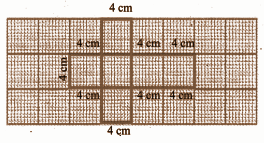# Samacheer Kalvi 8th Maths Book Solutions Term 1 Chapter 2 | Measurements Answers For Tamil Nadu Board

Samacheer Kalvi 8th Maths Book Solutions Term 1 Chapter 2 – Measurements are available here. The Samacheer Kalvi 8th Maths book answers of Term 1 Chapter 2, available at BYJU’S, contain step by step explanations designed by our mathematics experts. All these important questions are based on the new pattern prescribed by the Tamil Nadu board. Students can also get the solutions of other chapters on Samacheer Kalvi 8th Maths solutions. We have here, below in this article, the Samacheer Kalvi Class 8 Maths Book Term 1 Chapter 2 Questions and Solutions to solve and practice, so that students can face the exams more confidently.

## Samacheer Kalvi 8th Class Maths Textbook Term 1 Chapter 2 Questions With Solutions

Term 1 Chapter 2 of the Samacheer Kalvi 8th Maths guide will help the students to solve problems related to the parts of a circle, combined shapes, area of combined shapes, three-dimensional shapes, nets of three-dimensional shapes, the cross-section of solid shapes.

### Samacheer Kalvi 8th Maths Term 1 Chapter 2: Measurements Book Exercise 2.1 Questions and Solutions

Question 1: A circle of radius 120 m is divided into 8 equal sectors. Find the length of the arc of each of the sectors.

Solution:

The radius of the circle = 120m

Number of equal sectors = 8

The central angle of each sector = 360o / n

θ = 360o / 8

θ = 45o

Length of the arc = (θ / 360o) * 2𝛑r units

= [45o / 360o] * (2𝛑 * 120)

= 30𝛑 m

Question 2: In front of a house, flower plants are grown in a circular quadrant shaped pot whose radius is 2 feet. Find the area of the pot in which the plants grow. (𝛑 = 3.14).

Solution:

The central angle of the quadrant = 90o

The radius of the circle = 2 feet

Area of the quadrant = (θ / 360o) * 𝛑r2 units

= (90o / 360o) * 𝛑 * 2 * 2

= 3.14 sq.feet

Question 3: Dhamu fixes a square tile of 30 cm on the floor. The tile has a sector design on it as shown in the figure. Find the area of the sector. (𝛑 = 3 14).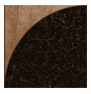Solution:

Side of the square = 30cm

The radius of the sector design = 30cm

Area of the quadrant = (1 / 4) 𝛑r2 sq.units

= (1 / 4) * (3.14) * (302)

= 706.5 cm2

### Samacheer Kalvi 8th Maths Term 1 Chapter 2: Measurements Book Exercise 2.2 Questions and Solutions

Question 1: Find the perimeter and area of the figure given below.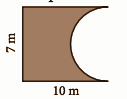Solution:

Length of the arc of the semi-circle = (1 / 2) * (2𝛑r) units

= (22 / 7) * (7 / 2)

= 11m

Perimeter = 11 + 10 + 7 + 10

= 38m

Area = Area of the rectangle – Area of semicircle

= (l * b) – (1 / 2) 𝛑r2

= (10 * 7) – (1 / 2) * (3.14) * (7 / 2)2

= 203 / 4

= 50.75 m2

Question 2: The doormat which is in a hexagonal shape has the following measures as given in the figure. Find its area.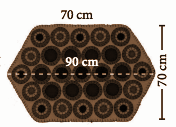Solution:

Area of the doormat = Area of 2 trapezium

Height of the trapezium = 70 / 2 = 35cm

a = 90cm, b = 70cm

Area of the trapezium = (1 / 2) * h * (a + b)

= (1 / 2) * (35) * (90 + 70)

= 5600cm2

Question 3: Find the area of the glass painting which has a triangle on a square

as given in the figure.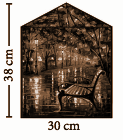Solution:

Area of the glass painting = area of the square + area of the triangle

Side of the square = a = 30cm

Base of the triangle = b = 30cm

Height of the triangle = h = 8cm

Area of the painting = a2 + (1 / 2) * b * h sq.units

= (30 * 30) + (1 / 2) * 30 * 8 cm2

= 1020 cm2

### Samacheer Kalvi 8th Maths Term 1 Chapter 2: Measurements Book Exercise 2.3 Questions and Solutions

Question 1: For each solid, three views are given. Identify for each solid, the corresponding top, front and side (T, F and S) views.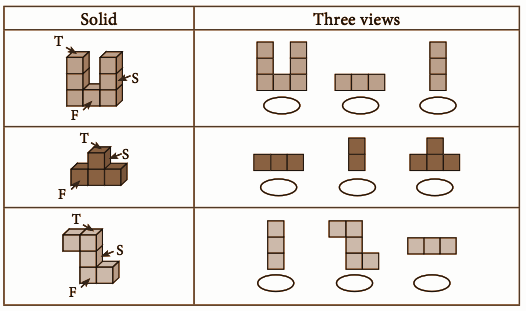Solution: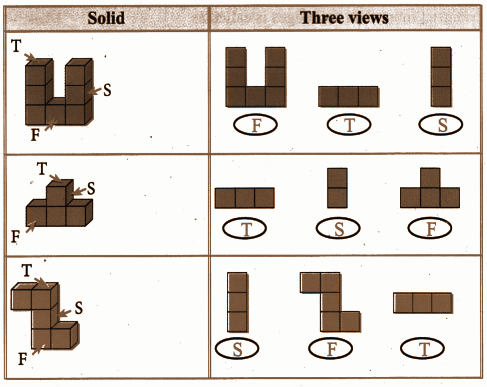Question 2: Which 3-D shapes do the following nets represent?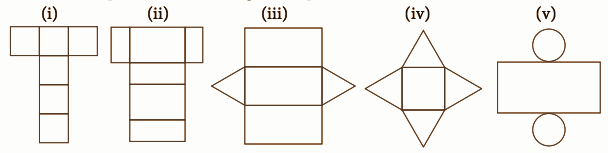Solution:

[i] The net represents a cube as it has 6 squares.

[ii] The net represents a cuboid.

[iii] The net represents a triangular prism.

[iv] The net represents a square pyramid.

[v] The net represents a cylinder.

Question 3: Can a polyhedron have 12 faces, 22 edges and 17 vertices?

Solution:

By Euler’s formula, F + V – E = 2 for a polyhedron.

F = 12, E = 22, V = 17

F + V – E = 12 + 17 – 22 = 7 ≄ 2

So, the polyhedron cannot have 12 faces, 22 edges and 17 vertices.

### Samacheer Kalvi 8th Maths Term 1 Chapter 2: Measurements Book Exercise 2.4 Questions and Solutions

Question 1: Find the area of the house drawing given in the figure.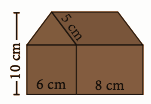Solution:

Area of the house = Area of the square + Area of the rectangle + Area of the triangle + Area of the parallelogram

= (6 * 6) + (8 * 6) + (0.5 * 6 * 4) + (8 * 4) cm2

= 36 + 48 + 12 + 32 cm2

= 128 cm2

Question 2: Draw the top, front and side view of the following solid shapes.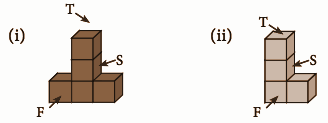Solution: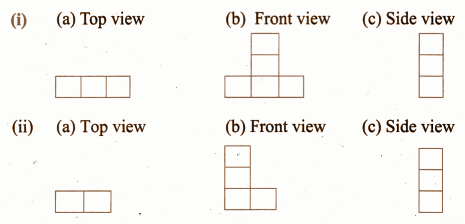Question 3: Draw the net for the cube of side 4 cm in a graph sheet.

Solution: